# ML Aggarwal Solutions for Class 7 Maths Chapter 14 Symmetry

ML Aggarwal Solutions for Class 7 Maths Chapter 14 Symmetry is the best study material for those students who are finding difficulties in solving problems. Our expert tutors formulate these exercises to assist you with your exam preparation to attain good marks in Maths. These solutions can help students clear doubts quickly and help in understanding the topic effectively. We suggest students who aim to score more in Maths practise ML Aggarwal Solutions for Class 7 Maths

Chapter 14 – Symmetry means that one shape becomes exactly like another when you move it in some way, turn, flip or slide. Topics covered in this ML Aggarwal Solutions for Class 7 Maths Chapter 14 are lines of symmetry for regular polygons, rotational symmetry, line symmetry and rotational symmetry.

## Download the PDF of ML Aggarwal Solutions for Class 7 Maths Chapter 14 Symmetry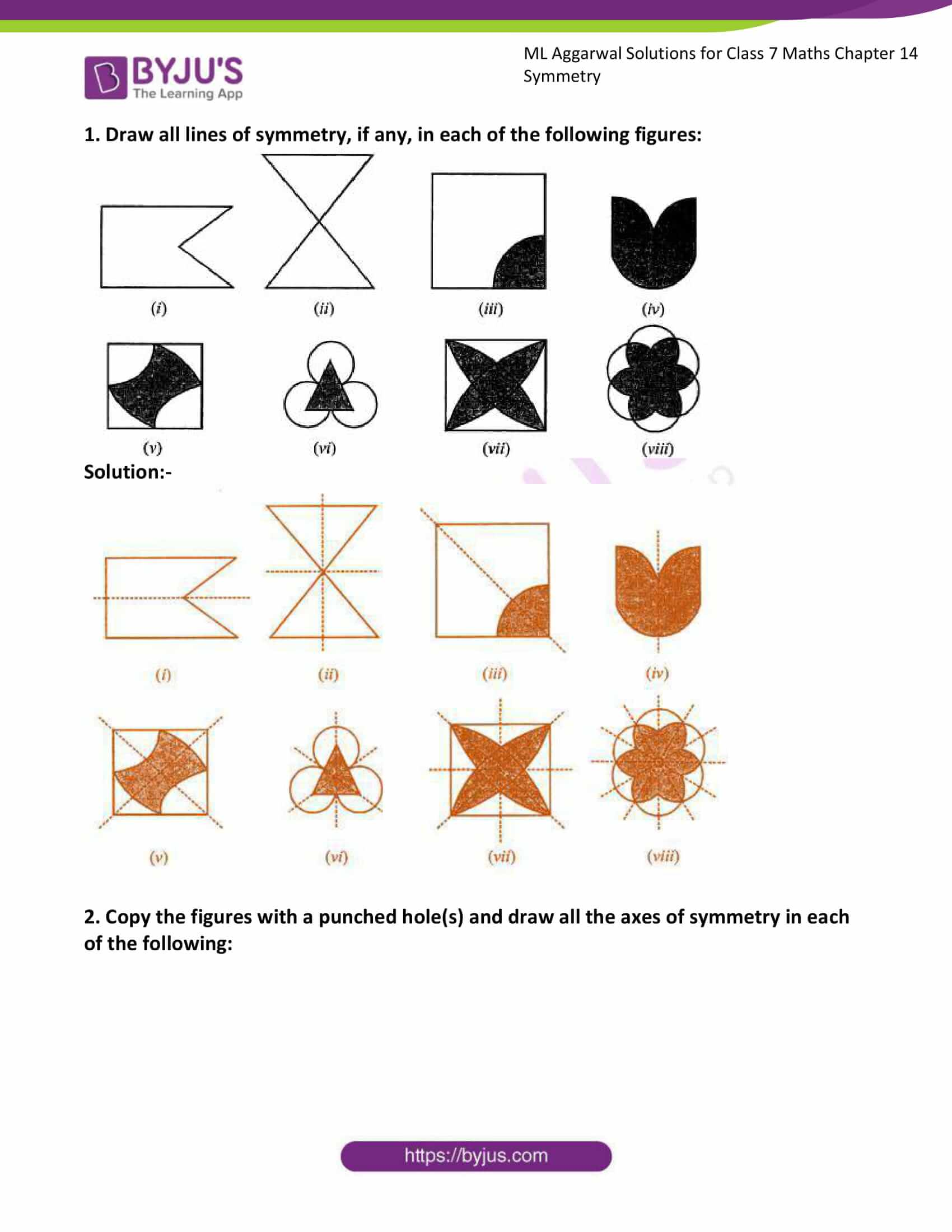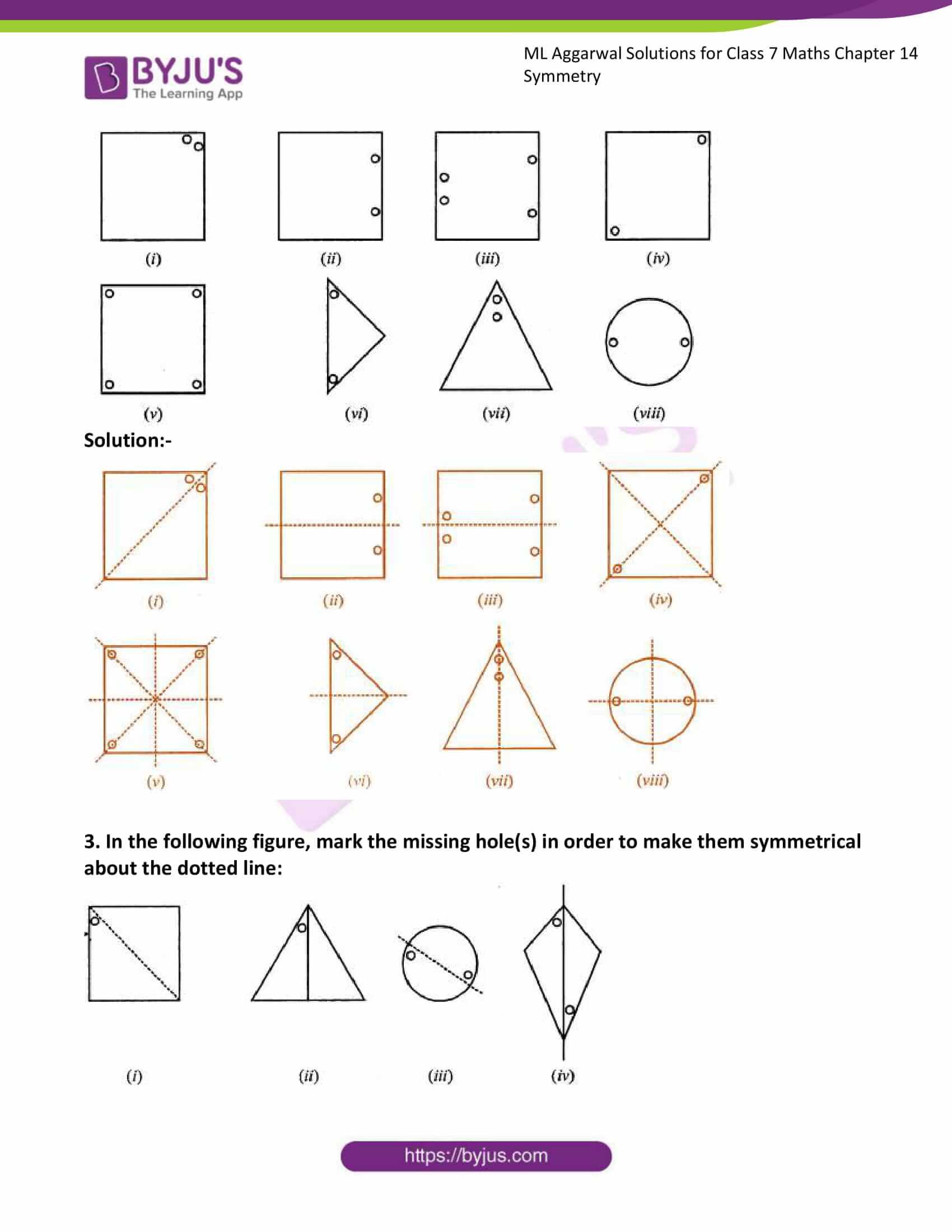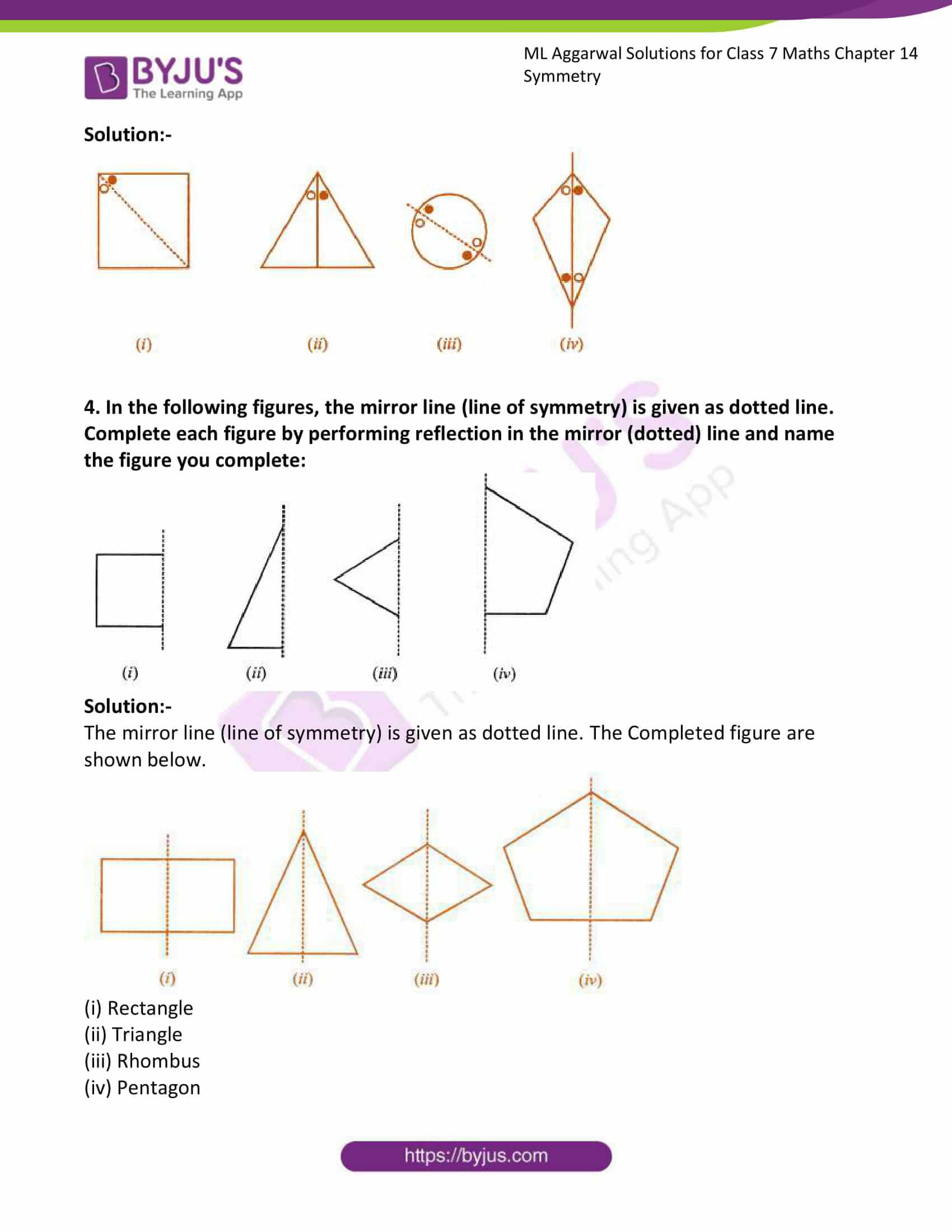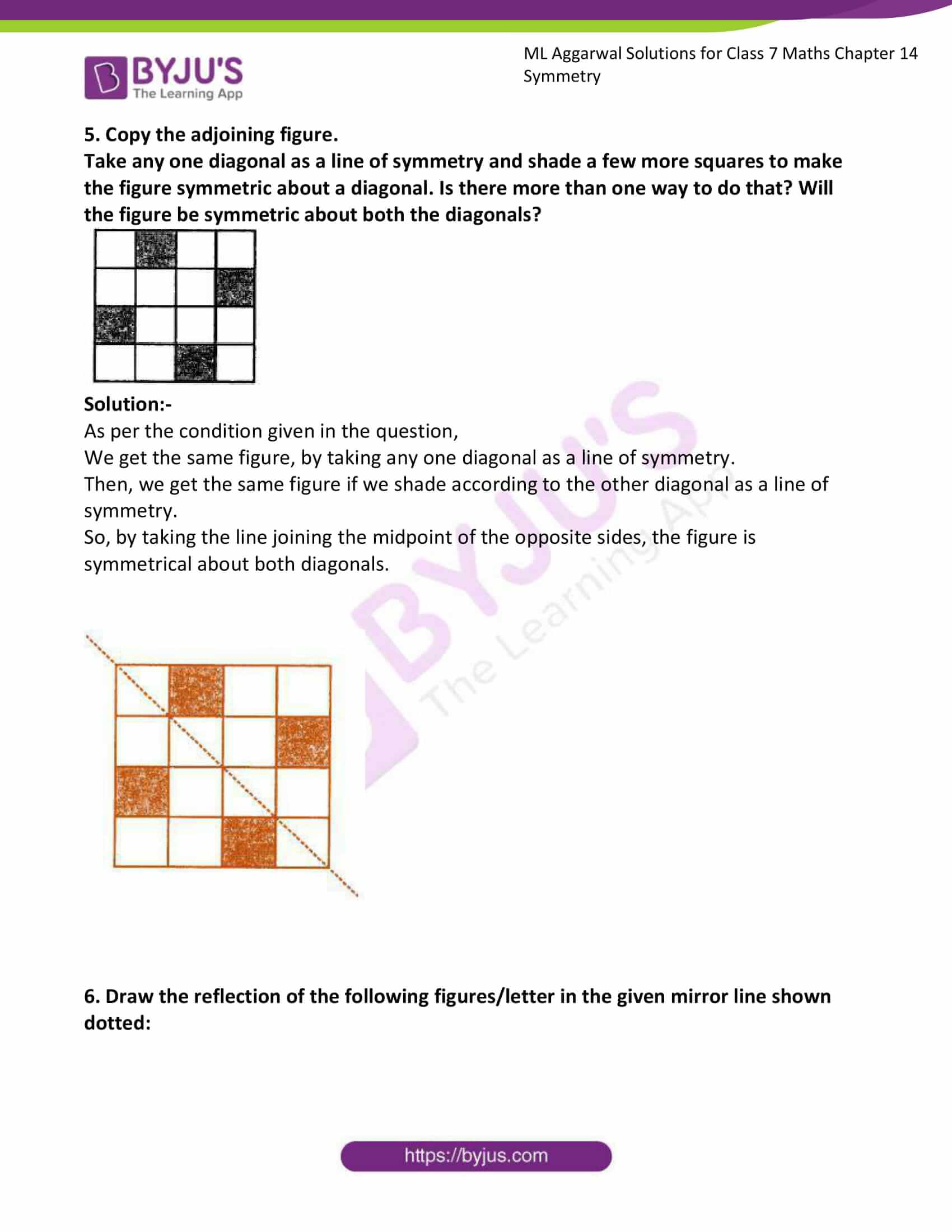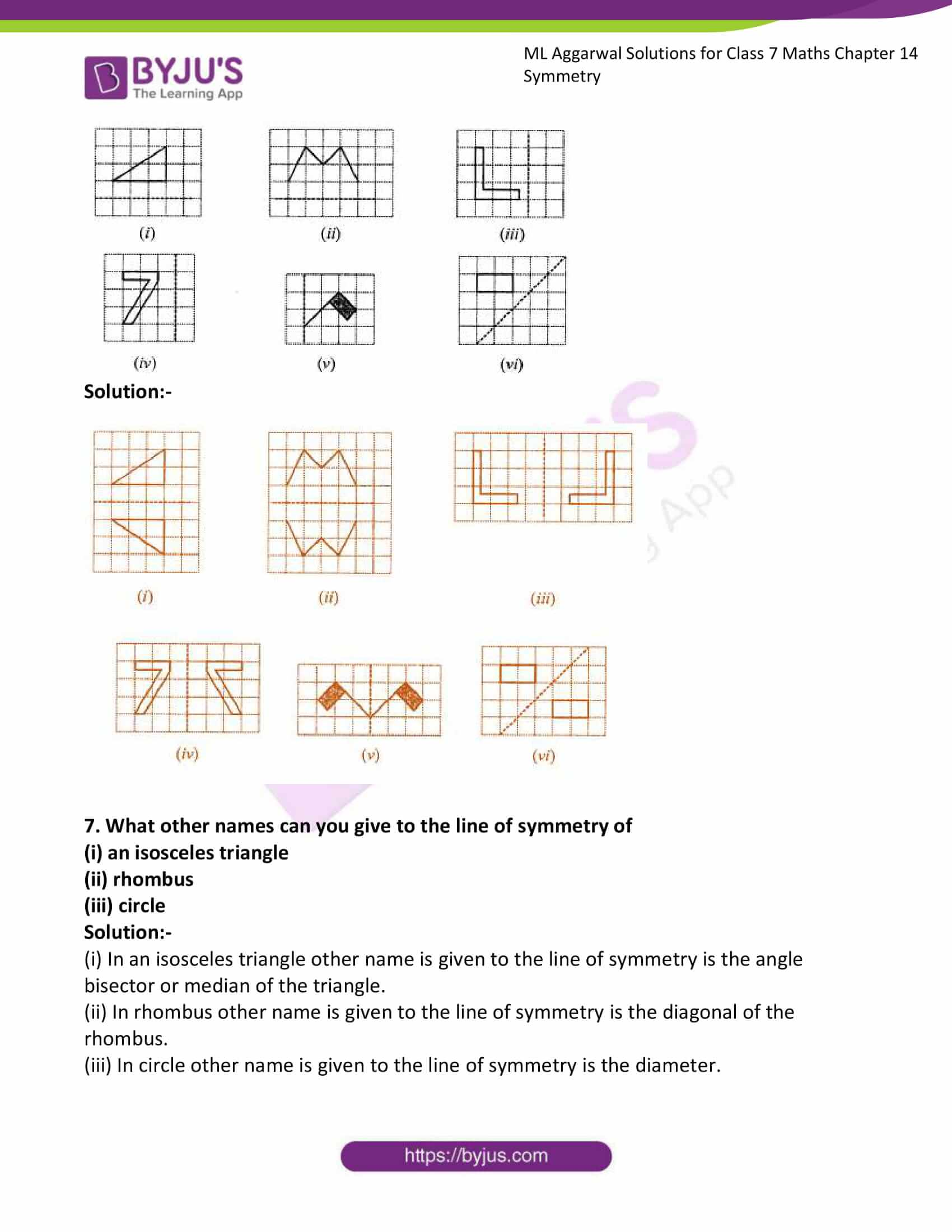### Access answers to ML Aggarwal Solutions for Class 7 Maths Chapter 14 Symmetry

1. Draw all lines of symmetry, if any, in each of the following figures: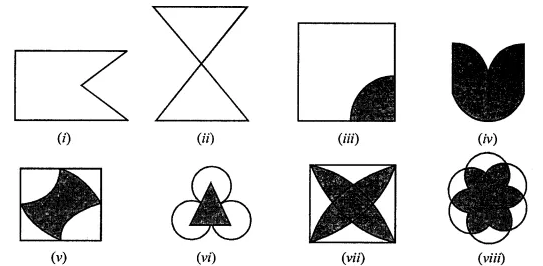Solution:-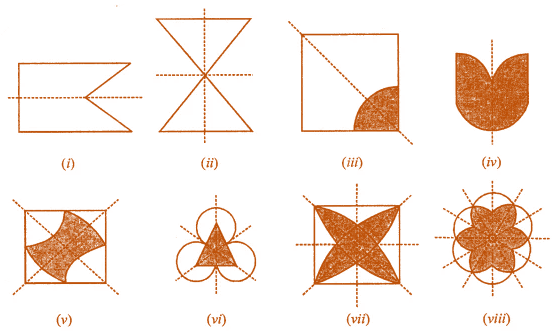2. Copy the figures with a punched hole(s) and draw all the axes of symmetry in each of the following: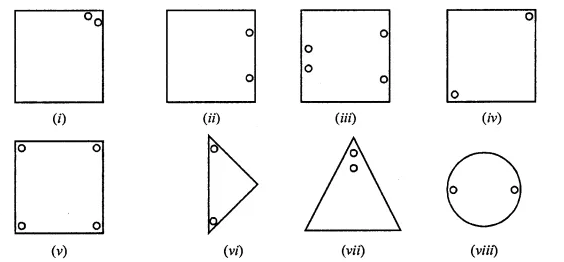Solution:-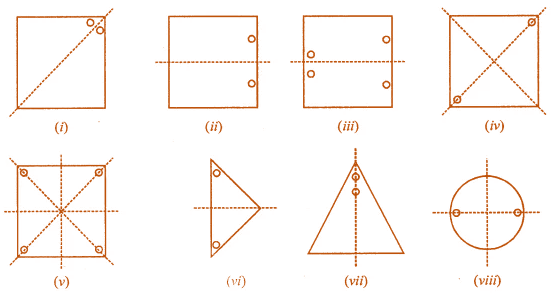3. In the following figure, mark the missing hole(s) in order to make them symmetrical about the dotted line: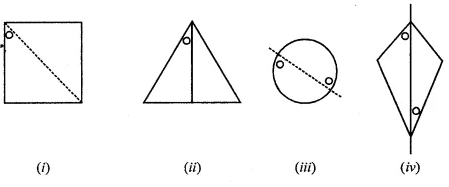Solution:-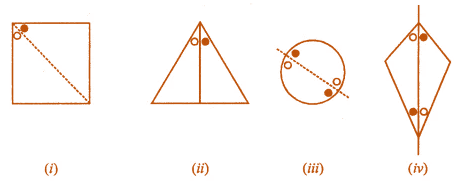4. In the following figures, the mirror line (line of symmetry) is given as dotted line. Complete each figure by performing reflection in the mirror (dotted) line and name the figure you complete: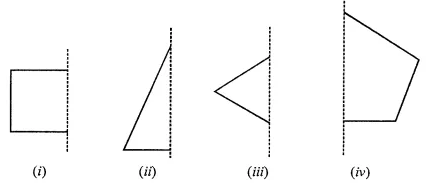Solution:-

The mirror line (line of symmetry) is given as dotted line. The Completed figure are shown below.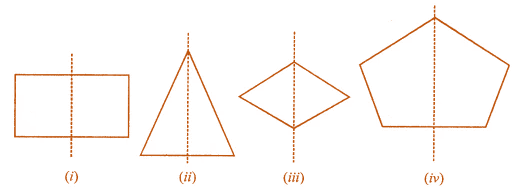(i) Rectangle

(ii) Triangle

(iii) Rhombus

(iv) Pentagon

Take any one diagonal as a line of symmetry and shade a few more squares to make the figure symmetric about a diagonal. Is there more than one way to do that? Will the figure be symmetric about both the diagonals?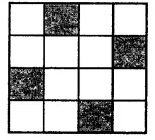Solution:-

As per the condition given in the question,

We get the same figure, by taking any one diagonal as a line of symmetry.

Then, we get the same figure if we shade according to the other diagonal as a line of symmetry.

So, by taking the line joining the midpoint of the opposite sides, the figure is symmetrical about both diagonals.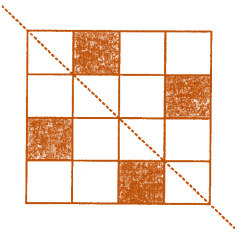6. Draw the reflection of the following figures/letter in the given mirror line shown dotted: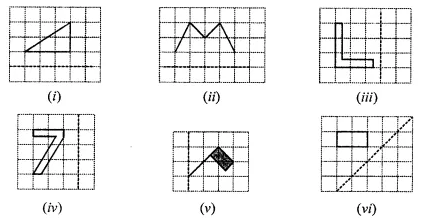Solution:-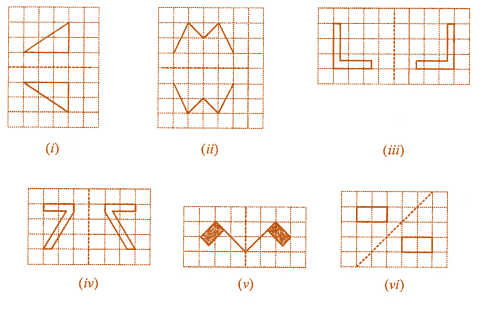7. What other names can you give to the line of symmetry of

(i) an isosceles triangle

(ii) rhombus

(iii) circle

Solution:-

(i) In an isosceles triangle other name is given to the line of symmetry is the angle bisector or median of the triangle.

(ii) In rhombus other name is given to the line of symmetry is the diagonal of the rhombus.

(iii) In circle other name is given to the line of symmetry is the diameter.